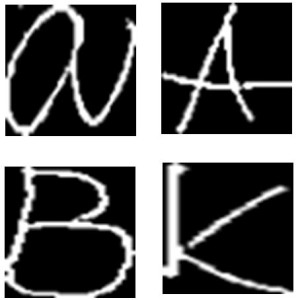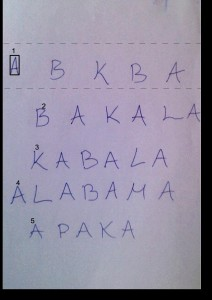Posted on

# Optical character recognition (OCR)Robert Cerny

Example down below shows conversion of scanned or photographed images of typewritten text into machine-encoded/computer-readable text. Process was divided into pre-processing, learning and character recognizing. Algorithm is implemented using the OpenCV library and C++.

## Â The process

1. Pre-processing â€“ grey-scale, median blur, adaptive threshold, closing
```cvtColor(source_image, gray_image, CV_BGR2GRAY);
medianBlur(gray_image, blur_image, 3);
adaptiveThreshold(blur_image, threshold, 255, 1, 1, 11, 2);
Mat element = getStructuringElement(MORPH_ELLIPSE, Size(3, 3), Point(1, 1));
morphologyEx(threshold, result, MORPH_CLOSE, element);
```

2. Learning â€“ we need image with different written styles of same character for each character we want to recognizing. For each reference picture we use these methods: findContours, detect too small areas and remove them from picture.
```vector < vector<Point> > contours;
vector<Vec4i> hierarchy;
findContours(result, contours, hierarchy, CV_RETR_CCOMP,
CV_CHAIN_APPROX_SIMPLE);
for (int i = 0; i < contours.size(); i = hierarchy[i]) {
Rect r = boundingRect(contours[i]);
double area0 = contourArea(contours[i]);
if (area0 < 120) {
drawContours(thr, contours, i, 0, CV_FILLED, 8, hierarchy);
continue;
}
}
```

Next step is to resize all contours to fixed size 50×50 and save as new png image.

```resize(ROI, ROI, Size(50, 50), CV_INTER_CUBIC);
imwrite(fullPath, ROI, params);
```

We get folder for each character with 50×50 images3. Recognizing â€“ now we know what look like A, B, C â€¦ For recognition of each character in our picture we use steps from previous state of algorithm. We pre-process our picture find contour and get rid of small areas. Next step is to order contours that we can easily output characters in right order.
```while (rectangles.size() > 0) {
vector<Rect> pom;
Rect min = rectangles[rectangles.size() - 1];
for (int i = rectangles.size() - 1; i >= 0; i--) {
if ((rectangles[i].y < (min.y + min.height / 2)) && (rectangles[i].y >(min.y - min.height / 2))) {
pom.push_back(rectangles[i]);
rectangles.erase(rectangles.begin() + i);
}
}
results.push_back(pom);
}
```Template matching is method for match two images, where template is each of our 50×50 images from learning state and next image is ordered contour.

```foreach detected in image
foreach template in learned
if detected == template
break
end
end
end
```

## Results

Recognizing characters with template matching in ordered contours array where templates are our learned images of characters. Contours images have to be resize to 51×51 pixels because our templates are 50×50 pixels.

```matchTemplate(ROI, tpl, result, CV_TM_SQDIFF_NORMED);
minMaxLoc(result, &minVal, &maxVal, &minLoc, &maxLoc, Mat());
if (maxVal >= 0.9) {	//treshold
cout << name; // print character
found = true;
return true;
}
```

Currently we support only characters A, B, K. We can see that character K was recognized twice from 4 characters in image.Â  That’s because out set of K written styles was too small (12 pictures). Recognition of characters A and B was 100 % successful (set has 120 written style pictures).